# 在数据结构与算法中 传值方式（C语言）# 传值方式

## 前言

``````Status InitList_Sq(SqList &L) {
//构造一个空的线性表L。
L.elem = (ElemType *)malloc(LIST_INIT_SIZE* sizeof(ElemType));
if(!L.elem)exit(OVERFLOW);
L.length=0;
L.listsize=LIST_INIT_SIZE;
return OK;
}
``````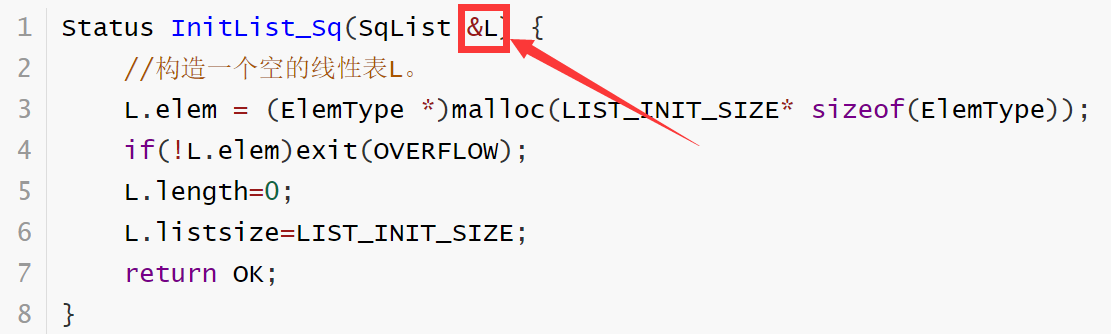``````Status GetElem(SqList L,int i,ElemType &e)   //这根本就不是取地址符
``````

``````Status GetElem(SqList L,int i,int &e)
``````

``````int *a,*b; /* 定义了两个整型的指针 */
int **a, **b; /* 定义了整型指针的指针 */
``````
``````//那么难道说是定义了整型变量为地址的变量e
int &e;
``````## C/C++中的引用参数

### 1.1 实参值不变

``````#include <stdio.h>
void swap(float m,float n)
{
float temp;
temp = m;
m = n;
n = temp;
}
void main()
{
float a,b;
printf ("please enter the number of a and b =");
scanf("%f %f",&a,&b);
swap (a,b);
printf ("the number of a = %f, the number of b = %f",a,b);
}
``````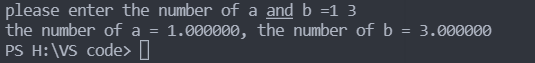### 1.2传地址方式——指针变量做参数，形参变化影响实参

``````#include <stdio.h>
void swap(float *m,float *n)
{
float temp;
temp = *m;      //取指针变量内容
*m = *n;
*n = temp;1
}
void main()
{
float a,b,*p1,*p2;
printf ("please enter the number of a and b =");
scanf("%f %f",&a,&b);
p1=&a;
p2=&b;
swap (p1,p2);
printf ("the number of a = %f, the number of b = %f",a,b);
}
````````````#include <stdio.h>
// 利用指针的经典解法
void swap(int *a, int *b)
{
int temp;
temp = *a;
*a = *b;
*b = temp;
}

void main()
{
int a = 1, b = 2;
swap(&a,&b);
printf("%d %d",a,b);
}
``````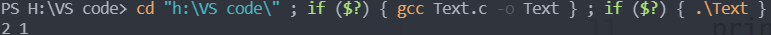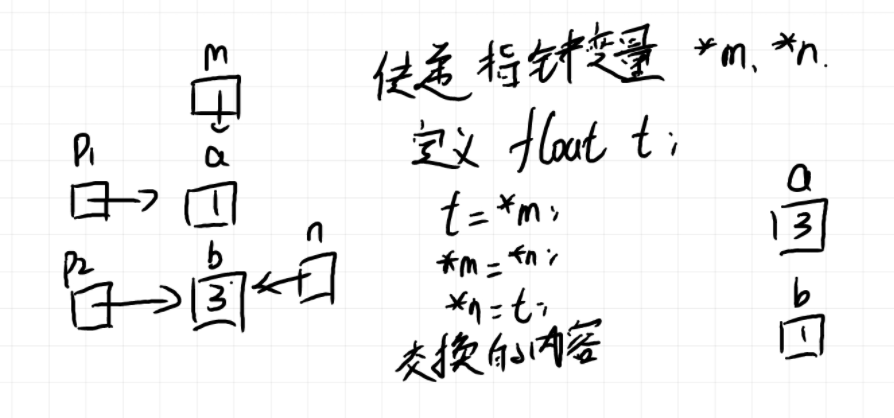### 形参变化不影响实参

``````#include <stdio.h>
void swap(float *a, float *b)
{
float *temp;
temp = a;
a = b;
b = temp;
}

void main()
{
float a = 1, b = 2;
swap(&a,&b);
printf("%f %f",a,b);
}
``````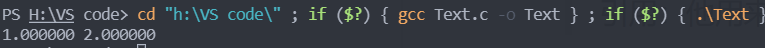### 1.4 传地址方式——数组名作参数

``````#include <stdio.h>
#include <string.h>
void sub(char *b)   //在C++语言中也可以使用b[]来写
{
strcpy(b,"world");
}
void main()
{
char a = "hello";
sub(a);  //传数组首地址
printf("%s",a);
}
``````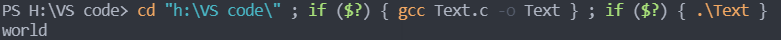### 1.5 传地址方式——引用类型做参数

``````//运用了C++的语法
#include <iostream>
#include <stdio.h>
// 引用参数实现交换
void swap(int &a, int &b){
int temp;
temp = a;
a = b;
b = temp;
}

// Using main to test
int main(){
int a = 1, b = 2;
swap(a,b);
printf("%d %d\n",a,b);
return 0;
}
``````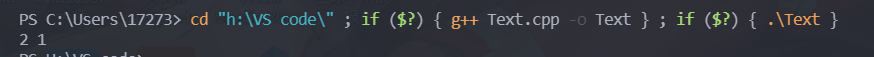## SqList *L的情况

### 最后

C语言不存在“引用”的概念，符号“&”只是表示取地址，而C++才有“引用”的含义。

``````//C语言不存在“引用”的概念，符号“&”只是表示取地址，而C++才有“引用”的含义。
//比如对于这段代码——
#include<stdio.h>
int main()
{
int i = 0;
int &j = i;
}，
/*使用gcc编译器编译会报错：”错误：expected identifier or ‘(’ before ‘&’ token“，但是使用g++编译就不会报错。*/
``````

1 total views,  1 views today